# Call Rate Settings

Call rate is used to charge the external calls made on the room phones.

System matches the called number with the call rate from top to bottom. Adjust the rate sequence by clicking these buttons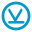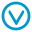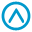.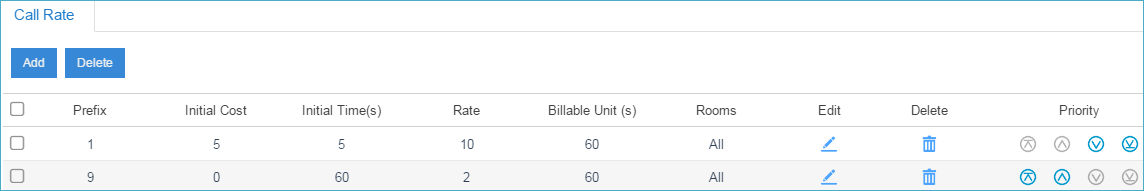## Call Cost

If a match is found then the cost is calculated as follows:

• Total Cost = Initial Cost + Billable Unit Number * Rate (less than one billable unit will be regarded as one billable unit)
• If the talking time is less than the “Initial Time”, the Total Cost = Initial Cost.

Below is an example billing rate setting, please check call cost details for different calls.

• Initial Time: 120 seconds
• Initial Cost: 0.2\$
• Rate: 0.3\$
• Billable Unit: 60 seconds
Table 1. Call Rate setting example
Talk Time (s) Total Cost (\$) Call Cost Details
68 0.2

Talk Time(68) < Initial Time(120)

Total Cost = Initial Cost

125 0.5

Talk Time: 125=120+5

Total Cost: 0.2+0.3*1=0.5

180 0.5

Talk Time: 180=120+60*1

Total Cost: 0.2+0.3*1=0.5

190 0.8

Talk Time: 190=120+60*1+10

Total Cost: 0.2+0.3*2=0.8

380 1.7

Talk Time: 380=120+4*60+20

Total Cost: 0.2+0.3*5=1.7

## Call Rate Settings

Go to Hotel > Settings > Call Rate to add or edit call rate.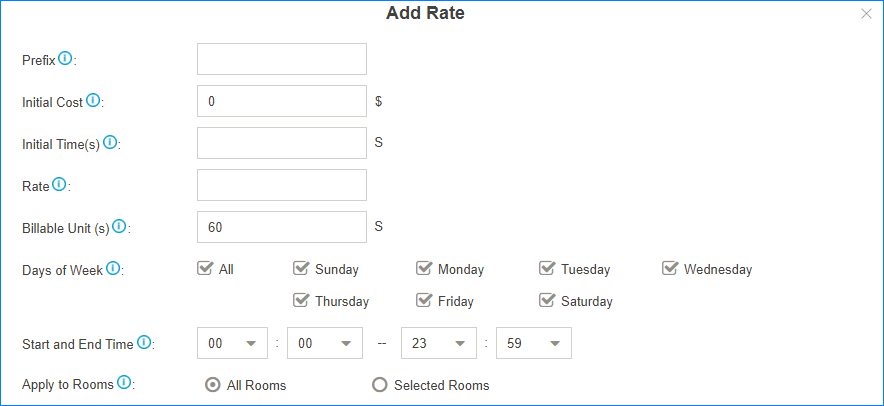• Prefix: Prefix of the called number. This setting must match the dial pattern of the outbound routes in your S-Series VoIP PBX.

Leave it blank, the rate will apply to all numbers.

• Initial Time& Initial Cost: If the Initial Cost is `\$0.2` and the Initial Time is `60` seconds, it means the first 60 seconds of this call will cost \$0.2.
• Rate: After the initial time, each billable unit will be charged with this rate.
• Billable Unit: Set the billable unit after initial time.

If the rate is \$0.2 and billable unit is 60 seconds, the call you make will cost \$0.2 per 60 seconds (less than one billing unit will be regarded as one billing unit).

• Days of Week: Set which days the rate will be applied to.
• Start and End Time: Set when the rate will be applied to.
• Apply to Rooms: Choose which room phones will use the call rate.